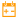# Date Math

 Block Group: Date Time Operations Icon:The Date Math block adds time to or subtracts time from the input date and time and returns the serial number that represents the result.

One use of the Date Math block is to calculate maturity dates. Another use is to calculate due dates that occur on the same day of the month as the date of issue.

For information on using dataflow blocks, see Dataflow.

## Input/Output Properties

The following properties of the Date Math block can take input and give output.

• input (number or date time string)
• op (enum)
• year (number)
• month (number)
• day (number)
• hour (number)
• minute (number)
• second (number)
• millisecond (number)

input specifies the serial number or any supported date time string as outlined here.

op specifies the operator that the Date Math block uses to perform calculations on the input date. The value of the op property can be one of the following:

• After: The values of the other inputs are added to the input value.
• Before: The values of the other inputs are subtracted from the input value.

year specifies the number of years to add or subtract, as a number with one to four digits.

month specifies the number of months to add or subtract.

day specifies the number of days to add or subtract.

hour specifies the number of hours to add or subtract.

minute specifies the number of minutes to add or subtract.

second specifies the number of seconds to add or subtract.

millisecond specifies the number of milliseconds to add or subtract.

## Output Property

The following property of the Date Math block can give output but cannot take input.

• output (number)

output returns the result of the operation.

## Examples

The following image shows two examples of the Date Math block. In the leftmost Date Math block, one month is added to the input date. In the rightmost Date Math block, one month, ten days, one hour, and five minutes are subtracted from the input date.